# 3. In Part A, you measure the absorbance of four FesCN" solutions and prepare a plot...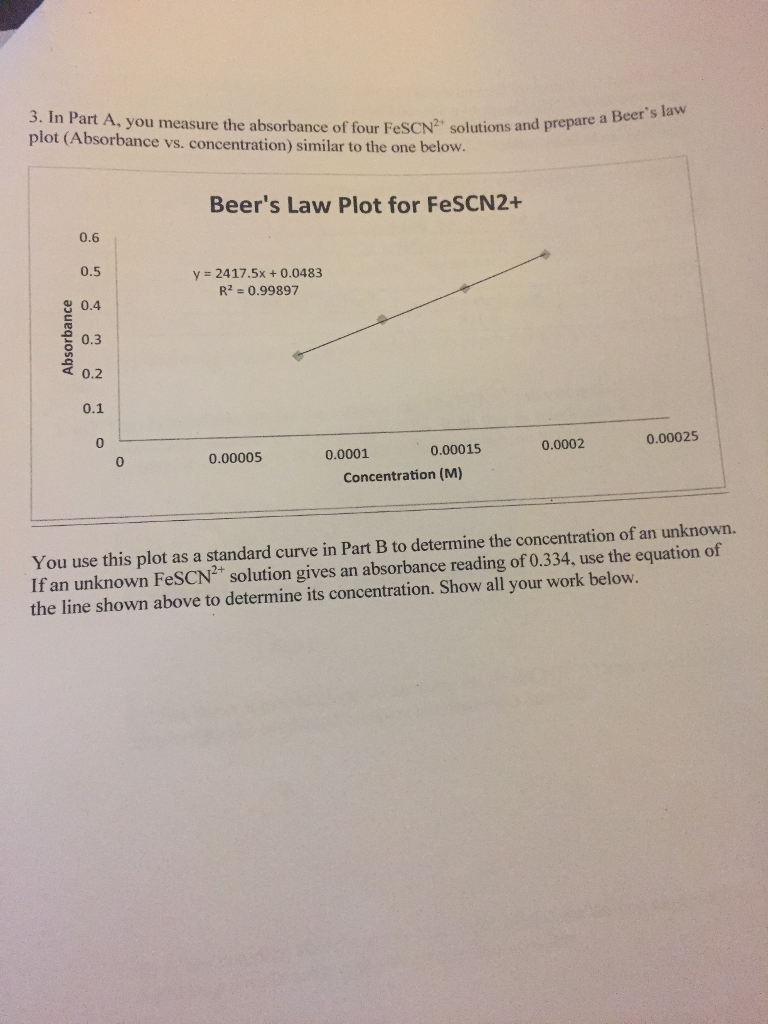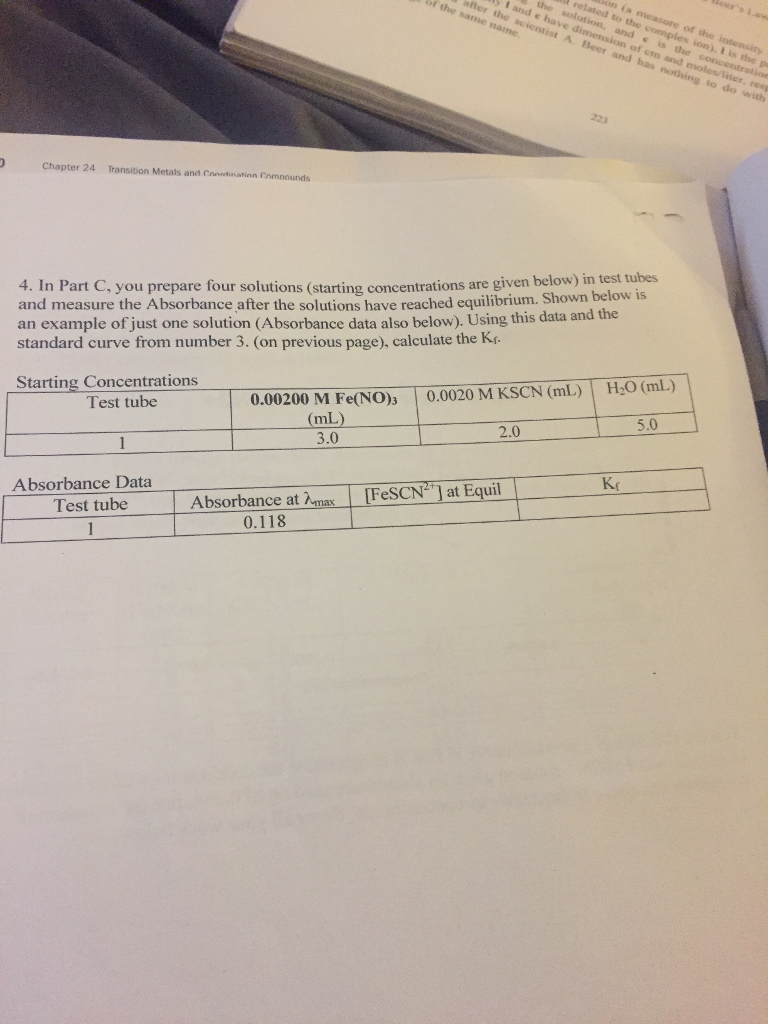3. In Part A, you measure the absorbance of four FesCN" solutions and prepare a plot (Absorbance vs. concentration) similar to the one below. Beer's Law Plot for FeSCN2 0.6 0.5 0.4 0.3 d 0.2 0.1 0 y = 2417.5× + 0.0483 R2 0.99897 0.00025 0.00015 0.0002 0.00005 0.0001 0 Concentration (M) You use this plot as a standard curve in Part B to determine the concentration of an unknown. If an unknown FeSCN2 solution gives an absorbance reading of 0.334, use the equation of the line shown above to determine its concentration. Show all your work below.

if

A = 0.334

then

A = 2417.5*C + 0.0483

0.334= 2417.5*C + 0.0483

solve for C

C = (0.334-0.0483)/2417.5

C = 0.000118 M

[FeSCN+2] = 0.000118 M

Q4

Kf = [FeSCN+2]/[Fe+3][SCN-]

initially

[Fe+3] = M1V1/(Vtotal) = 0.002*3/(3+2+5) = 0.0006

[SCN-] = M1V1/(Vtotal) = 0.002*2/(3+2+5) = 0.0004

[FeSCN+2] = 0

in equilbirium

[Fe+3] = = 0.0006-x

[SCN-] = = 0.0004 - x

[FeSCN+2] = x

get x

A = 2417.5*C + 0.0483

0.118 = 2417.5*C + 0.0483

C =( 0.118 -0.0483)/2417.5 = 0.0000288 M

[Fe+3] = = 0.0006-0.0000288 = 0.0005712

[SCN-] = = 0.0004 - 0.0000288 = 0.0003712

[FeSCN+2] = 0.0000288

substitute in Kf

Kf = (0.0000288 )/(0.0003712*0.0005712 )

Kf = 135.83

##### Add Answer to: 3. In Part A, you measure the absorbance of four FesCN" solutions and prepare a plot...
Similar Homework Help Questions
• ### Keq determination 1 0.9 2900X 0.00 R -0.9985 08 Absorbance 0.7 0.6 0.5 0.4 0.3 0.2...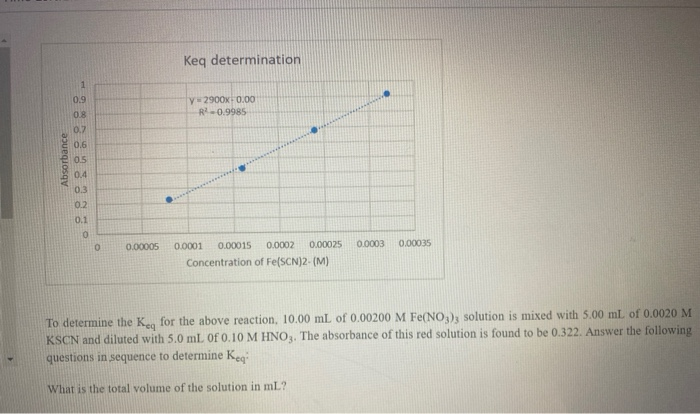Keq determination 1 0.9 2900X 0.00 R -0.9985 08 Absorbance 0.7 0.6 0.5 0.4 0.3 0.2 0.1 0 0.00005 0.0003 0 0.00035 0.0001 0.00015 0.0002 0.00025 Concentration of Fe(SCN)2-(M) To determine the Key for the above reaction, 10.00 mL of 0.00200 M Fe(NO3); solution is mixed with 5.00 ml of 0.0020 M KSCN and diluted with 5.0 ml of 0.10 M HNO3. The absorbance of this red solution is found to be 0.322. Answer the following questions in sequence to...

• ### need help. please explain Mixture FeSCN Absorbance O-149 O-250 O-314 O.432 O.521 1 3 Caloudations xperiment...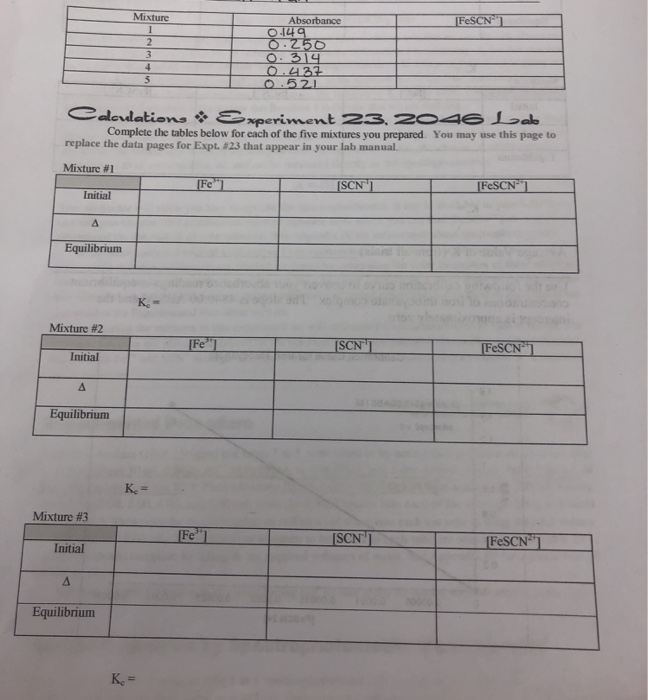need help. please explain Mixture FeSCN Absorbance O-149 O-250 O-314 O.432 O.521 1 3 Caloudations xperiment 23. z046 Lab Complete the tables below for each of the five mixtures you prepared. You may use this page to replace the data pages for Expt. # 23 that appear in your lab manual. Mixture #1 [Fe ISCN [FeSCN1 Initial A Equilibrium K Mixture #2 [Fe"] SCNT FESCN Initial Equilibrium Ke= Mixture #3 Fe"] SCN' FESCN Initial Equilibrium K.= Mixture #4 FeSCN Fe...

• ### I need the whole table to be completed and calculations only for E2 Beer's Law Plot...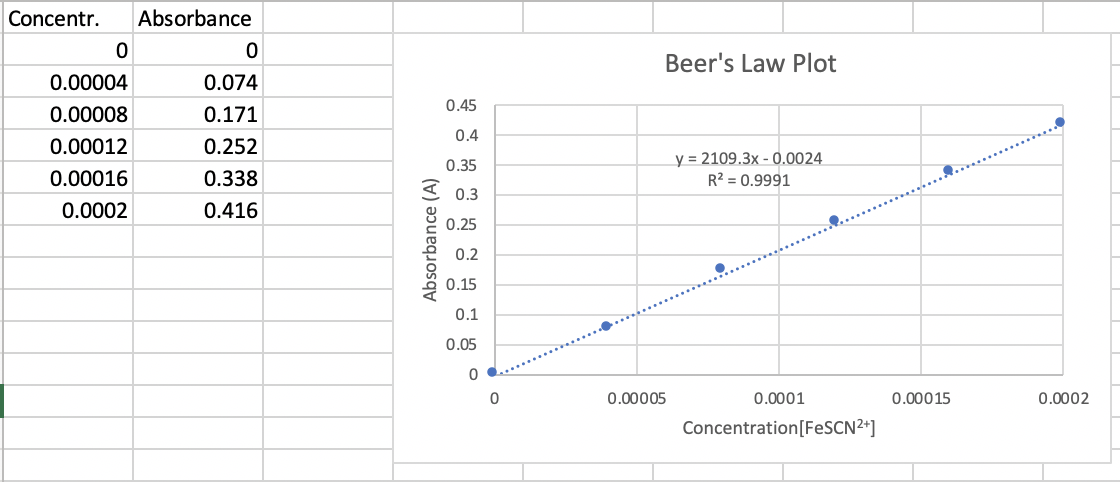I need the whole table to be completed and calculations only for E2 Beer's Law Plot 0.45 Concentr. Absorbance 0 0 0.00004 0.074 0.00008 0.171 0.00012 0.252 0.00016 0.338 0.0002 0.416 0.4 0.35 I 0,3 y = 2109.3x -0.0024 R2 = 0.9991 0.25 Absorbance (A) 0.2 0.15 0.1 0.05 0 0 0.00005 0.0001 0.00015 0.0002 Concentration(FeSCN2+] Part II. Equilibrium Mixtures Stock solution concentration, [NaSCN) = 0.00200 M (in 0.10 M HNO3) Stock solution concentration, [Fe(NO3)3] = 0.00200 Min 0.10 M...

• ### Here are the graphs. instrument should have an absorbance reading of zero when there is no...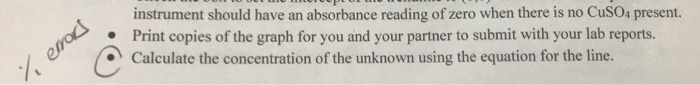Here are the graphs. instrument should have an absorbance reading of zero when there is no CuS04 present. Print copies of the graph for you and your partner to submit with your lab reports. Calculate the concentration of the unknown using the equation for the line. emros Concentration (M) Absorbance 0.5 1.005 0.3 0.704 0.617 0.25 0.1 0.291 0,05 0.131 0.513 Concentration VS Absorbance 1.2 y 2.1773x R2 0.9566 1 e4e4eeresases4s4 0.8 0.6 0.4 0.2 0.4 0.5 0.6 0.1 0.2...

• ### Beers law solve for E3 and E4 showing work Part II. Equilibrium Constant Calculations Calculate the...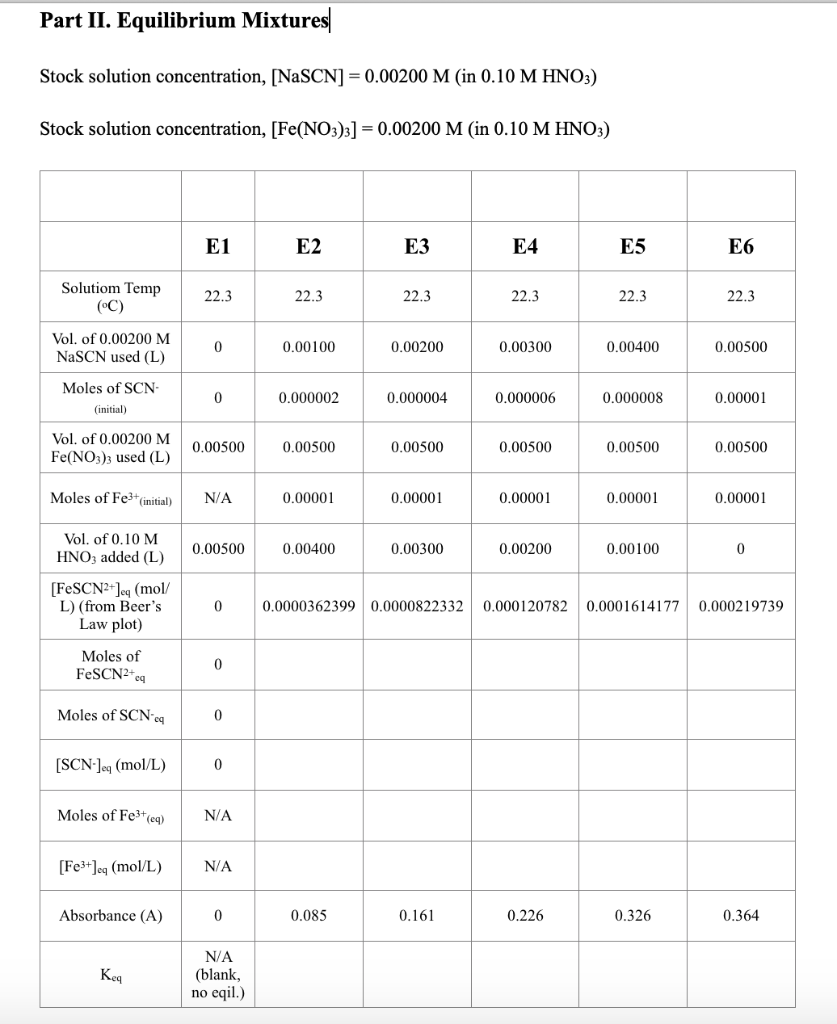Beers law solve for E3 and E4 showing work Part II. Equilibrium Constant Calculations Calculate the initial moles of Fe3+ and SCN- for solutions E2 through E6 and record these values in the table on your Data Sheet. You obtain the moles of the reactants by multiplying their molarity by the volume (in L) of their solution used. Determine the equilibrium concentration of FeSCN2+ for each of the solutions E2-E6 from your Beer’s Law plot. Using these equilibrium concentrations, the...

• ### For the solutions that you will prepare in Step 2 of Part I below, calculate the [FeSCN2 Presume ...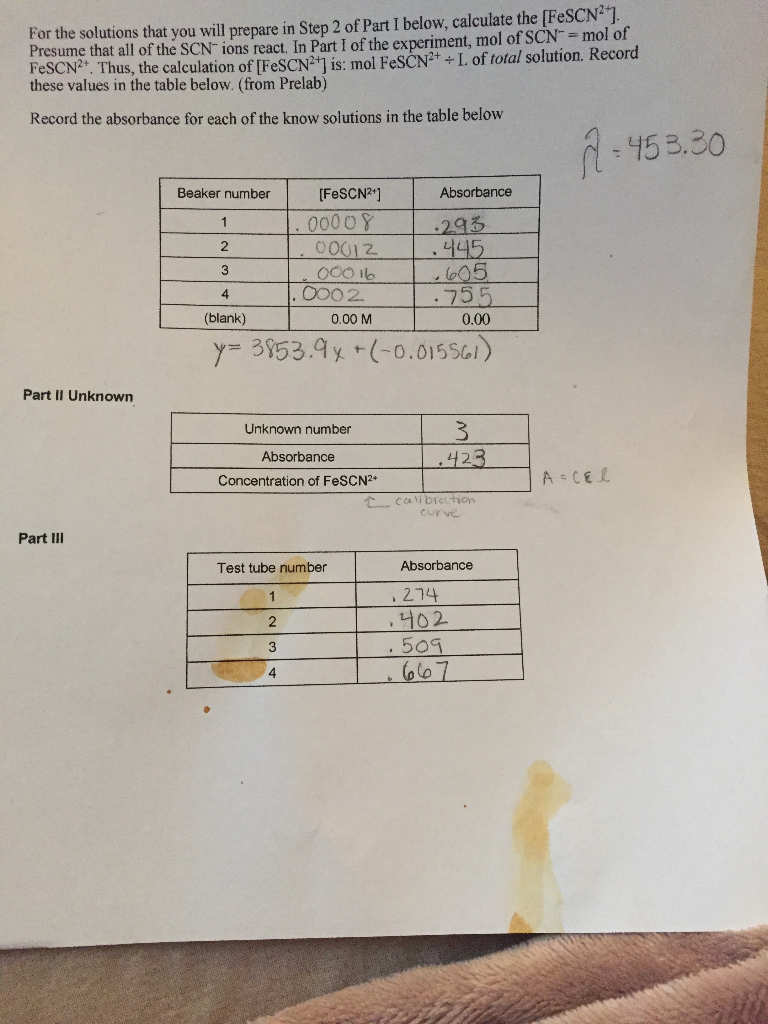Please help me fill out Part III For the solutions that you will prepare in Step 2 of Part I below, calculate the [FeSCN2 Presume that all of the SCN-ions react, In Part I of the experiment, mol of SCN mol of FeSCN2+. Thus, the calculation of [FeSCN2+1 is: mol FeSCN2+L of total solution. Record these values in the table below. (from Prelab) Record the absorbance for each of the know solutions in the table below 453.30 Beaker number FeSCN2*]...

• ### 2. In Part A of this experiment, you prepare five FeSCN solutions (one that is just...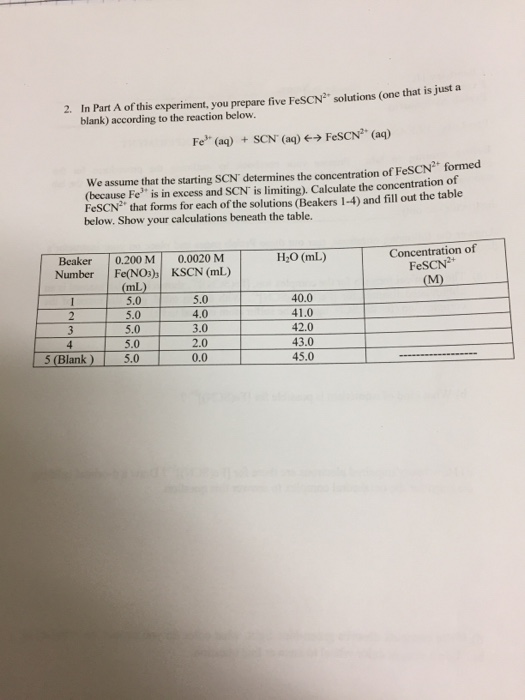2. In Part A of this experiment, you prepare five FeSCN solutions (one that is just a blank) according to the reaction below Fe (aq) SCN (aq) > FeSCN (a) SCN formed We assume that the starting SCN determines the concentration of Fe (because Fe is in excess and SCN is limiting). Calculate the concentration ot FeSCN2 that forms for each of the solutions (Beakers 1-4) and fill out the table below. Show your calculations beneath the table. Concentration of...

• ### Concentration v. Absorbance

A 3 mg/ml stock solution was diluted as described in the table below to make a standard curve vs absorbance. An unknown sample has an absorbance of 0.64. What isits approximate concentration?InstructorsVolume of Stock solution (3 mg/ml)Volume of waterAbs5 ml0 ml1.004 ml1 ml0.83 ml2 ml0.62 ml3 ml0.41 ml4 ml0.20 ml5 ml0.0

• ### please do 1 through 4. Thank you. 0 1. Fe 1.SCN Procedure B: 1. Prepare ICE...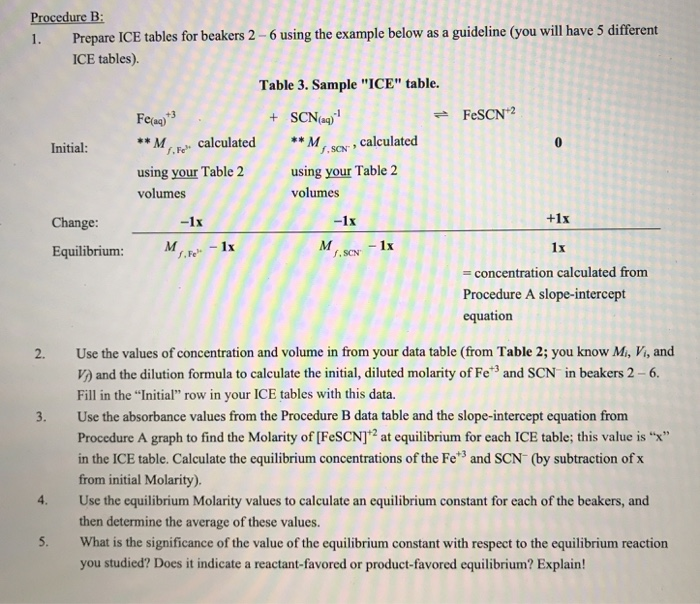please do 1 through 4. Thank you. 0 1. Fe 1.SCN Procedure B: 1. Prepare ICE tables for beakers 2 - 6 using the example below as a guideline (you will have 5 different ICE tables). Table 3. Sample "ICE" table. Fe(aq)*3 + SCN(aq) = FeSCN2 Initial: **M calculated **M calculated using your Table 2 using your Table 2 volumes volumes Change: - 1x - 1x Equilibrium: M 1x 1x = concentration calculated from Procedure A slope-intercept equation +1x M...

• ### 6. (20 points) A Beer's law curve for a red food dye (max ed food dye...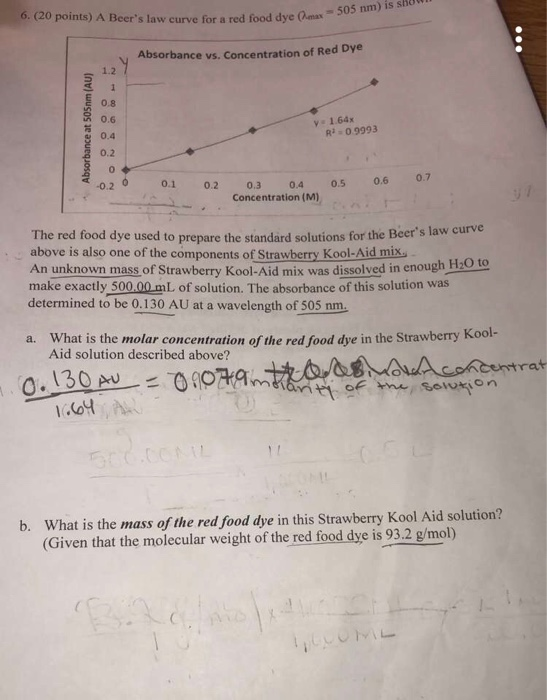6. (20 points) A Beer's law curve for a red food dye (max ed food dye (max = 505 nm) is shown Absorbance vs. Concentration of Red Dye Absorbance at 505nm (AU) NO NON y 1.64x R1 -0.9993 0.1 0.2 0.5 0.6 0.7 0.3 0.4 Concentration (M) The red food dye used to prepare the standard solutions for the Beer stay above is also one of the components of Strawberry Kool Aid mix. An unknown mass of Strawberry Kool-Aid mix...

Free Homework App